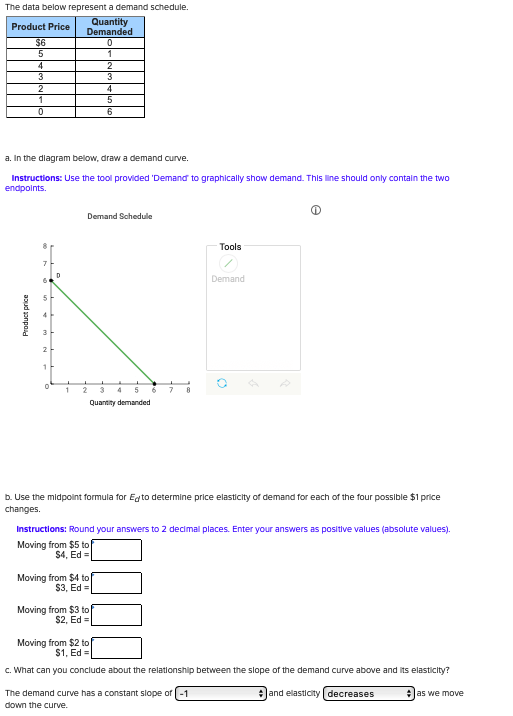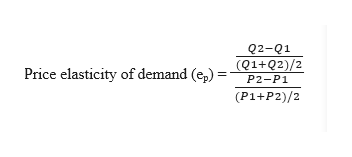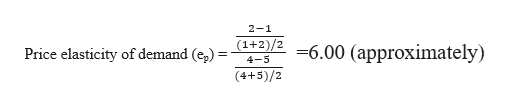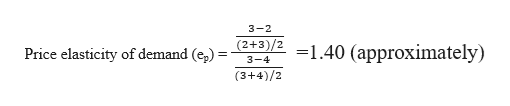The data below represent a demand schedule.QuantityDemandedProduct Price\$6C543341506a. In the diagram below, draw a demand curveInstructions: Use the tool provided Demand to graphically show demand. This line should only contain the twoendpointsDemand ScheduleToolsDemand57Quantity demandedb. Use the midpoint formula for Eqto determine price elasticity of demand for each of the four possible \$1 pricechangesInstructions: Round your answers to 2 decimal places. Enter your answers as positive values (absolute valuesMoving from \$5 to\$4, EdMoving from \$4 toS3, EdMoving from \$3 toS2, EdMoving from \$2 to\$1, Edc. What can you conclude about the relationship between the slope of the demand curve above and its elasticity?The demand curve has a constant slope of-1and elasticity decreasesas we movedown the curve.eaud onpod

Question

i just want part B done, not the rest of it.help_outlineImage TranscriptioncloseThe data below represent a demand schedule. Quantity Demanded Product Price \$6 C 5 4 3 3 4 1 5 0 6 a. In the diagram below, draw a demand curve Instructions: Use the tool provided Demand to graphically show demand. This line should only contain the two endpoints Demand Schedule Tools Demand 5 7 Quantity demanded b. Use the midpoint formula for Eqto determine price elasticity of demand for each of the four possible \$1 price changes Instructions: Round your answers to 2 decimal places. Enter your answers as positive values (absolute values Moving from \$5 to \$4, Ed Moving from \$4 to S3, Ed Moving from \$3 to S2, Ed Moving from \$2 to \$1, Ed c. What can you conclude about the relationship between the slope of the demand curve above and its elasticity? The demand curve has a constant slope of-1 and elasticity decreases as we move down the curve. e aud onpod fullscreen
Step 1

The mid-point formula for Price elasticity of demand:help_outlineImage TranscriptioncloseQ2-Q1 Price elasticity of demand (e,) =~Q1+Q2)/2_ P2-P1 (P1+P2)/2 fullscreen
Step 2

Moving from P1=\$5 to P2=\$4, Q1=1 and Q2=2

Ignoring the negative sign, when (ep) >1, its an elastic demand.help_outlineImage Transcriptionclose2-1 Price elasticity of demand (e,) = /2 =6.00 (approximately) 4-5 (4+5)/2 fullscreen
Step 3

Moving from P1=\$4 to P2=\$3, Q1=2 and Q2=3

Ignoring the negative si...help_outlineImage Transcriptionclose3-2 Price elasticity of demand (e,) = */ =1.40 (approximately) 3-4 (3+4)/2 fullscreen

Want to see the full answer?

See Solution

Want to see this answer and more?

Our solutions are written by experts, many with advanced degrees, and available 24/7

See Solution
Tagged in